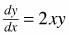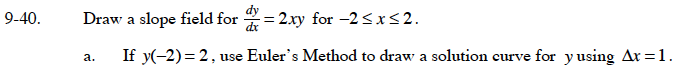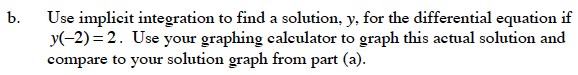### Home > CALC > Chapter 9 > Lesson 9.1.3 > Problem9-40

9-40.
1. Draw a slope field forfor −2 ≤ x ≤ 2. Homework Help ✎

1. If y(−2) = 2, use Euler's Method to draw a solution curve for y using ∆x = 1.

2. Use implicit integration to find a solution, y, for the differential equation if y(−2) = 2. Use your graphing calculator to graph this actual solution and compare to your solution graph from part (a).If dx = 1, then dy = 2xy.
The initial point is (−2, 2).
The next point is (–2 + dx, 2 + dy).Use separation of variables and the integrate.